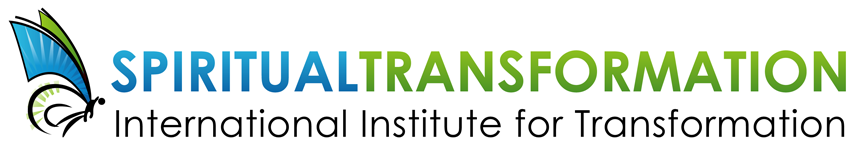# JULY 2019

## Summer is for contemplation ... and fun!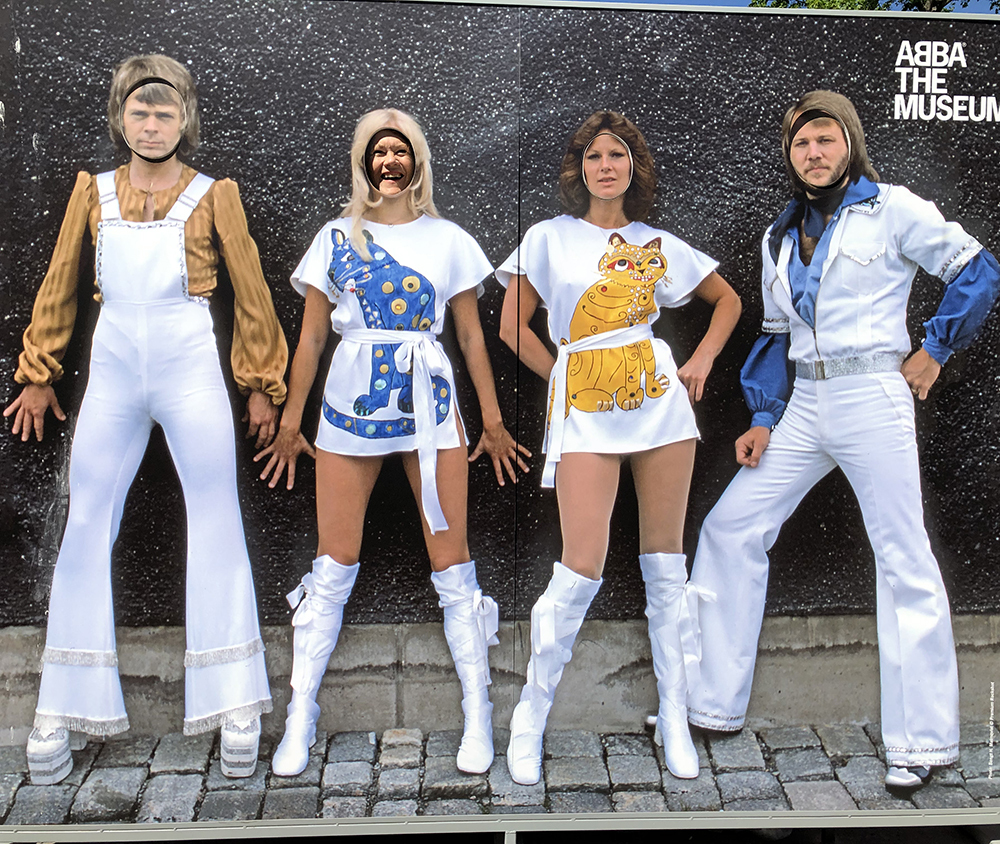Guess who the new ABBA member is!

Dear Friend,

Summer is the perfect time to get away from it all and recharge. But if you don't have time for this, I'm offering a very special summer sale on ALL of my CDs and MP3 downloads for the month of July! See below for details and to order. And view my brand new Youtube video which provides more details on the recording, Rise of the Unconscious: Your Basic Goodness.

I'm so pleased to be able to announce that we will be launching a new self-study e-course this fall, Ancestor and Family Healing. Details coming soon.

Enjoy some favorite memories with my photo album from European workshops this past June.

This will be the last call for my Diamond Heart Meditation Retreat, located on the sea coast north of Vancouver, BC. There's only one spot left!

 table div table+table+table+table+table div table{width:100%;padding:0}table div table+table+table+table+table div table img{width:96.23%;padding:0;float:none}table div table+table+table+table+table div table td{width:100%;padding:0 1.88% 18px}/* styles *//* styles */
 table div table+table+table+table+table+table+table div table{width:100%;padding:0}table div table+table+table+table+table+table+table div table img{width:96.23%;padding:0;float:none}table div table+table+table+table+table+table+table div table td{width:100%;padding:0 1.88% 18px}/* styles */## NEW VIDEO: Rise of the Unconscious

 /* styles */ We can all be true to our authentic selves - our basic goodness - if we work at it. Learn how to stop being so self-critical! In this all new video, I discuss how this audio recording can help you achieve heart opening and love for yourself and others as you joyfully discover you are wonderful just as you are. This audio recording, Rise of the Unconscious, describes the steps we go through to unite our personal and collective unconscious to be our authentic selves—a soul-infused personality. View the video on Youtube here: https://youtu.be/NA8p0oi82Zo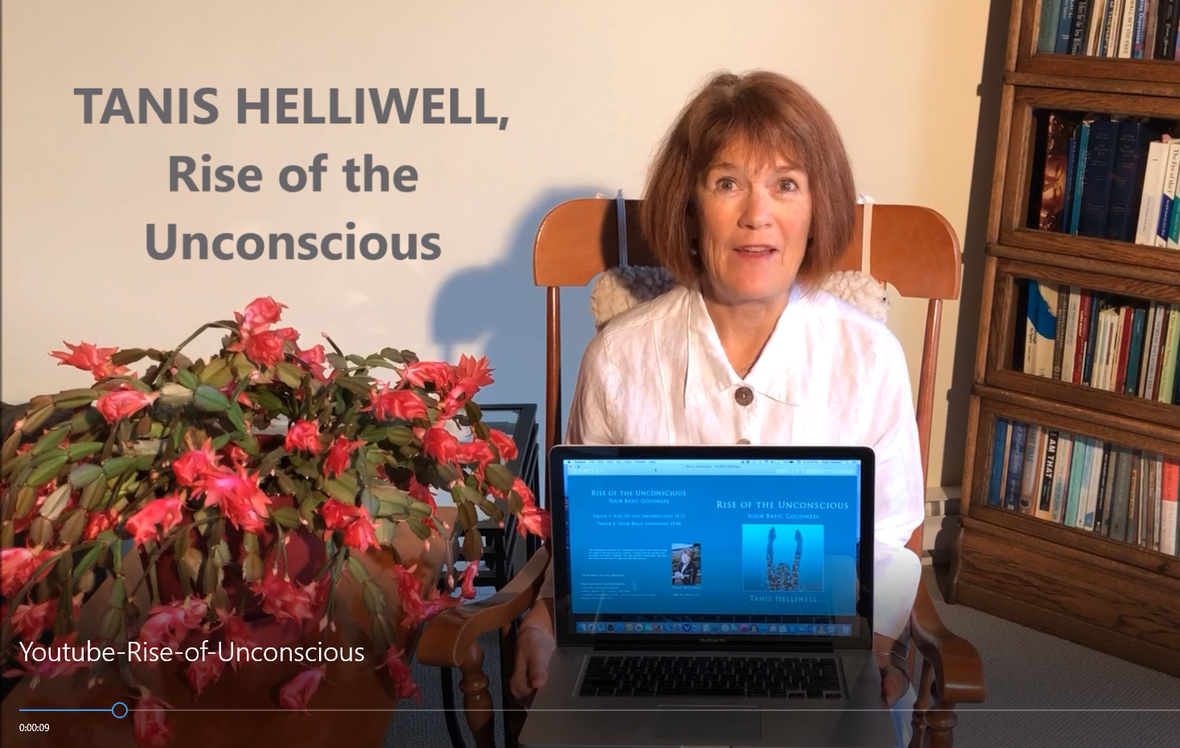table div table+table+table+table+table+table+table+table+table+table+table div table{width:100%;padding:0}table div table+table+table+table+table+table+table+table+table+table+table div table img{width:96.23%;padding:0;float:none}table div table+table+table+table+table+table+table+table+table+table+table div table td{width:100%;padding:0 1.88% 18px}/* styles */# All CDs \$9 each | MP3s \$5.95 each -- for July only

(Regular prices \$14.95 & \$9.95)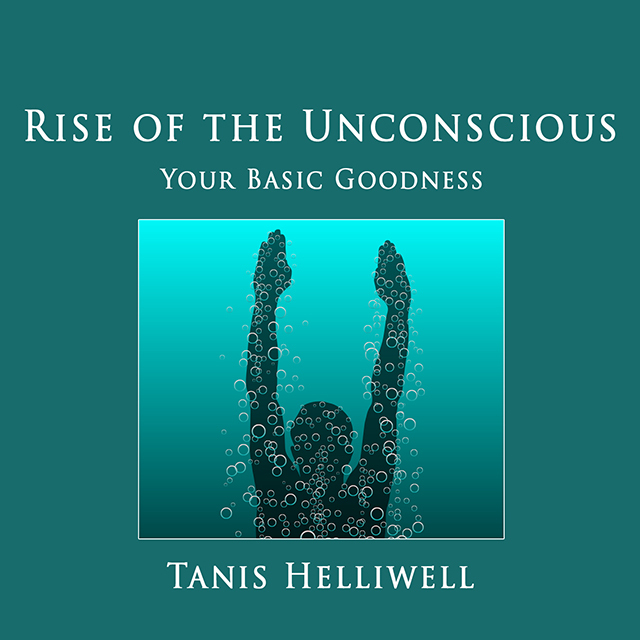To help folks in inner contemplation and meditation this summer, all audio recordings are on sale for the month of July!

As described in my new video above (for example), my recording, Rise of the Unconscious, helps you make sense of the WHY our world is in chaos currently so you can see the positive outcome from it.

Allow us to assist you on your spiritual transformation journey this summer. Purchase Rise of the Unconscious as a CD or an MP3, OR download the entire Self-Healing Series of audio recordings for \$15 (regular price \$20)!

The Self-Healing series includes: Rise of the Unconscious, Reawakening Ancestral Memories and my most popular recording, The Body Elemental: Healing with Your Body Elemental.

Purchase individual recordings HERE.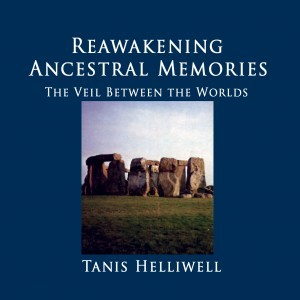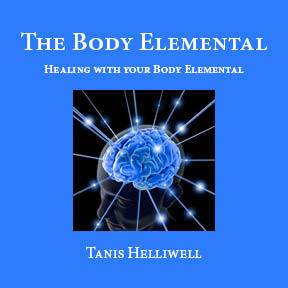table div table+table+table+table+table+table+table+table+table+table+table+table+table+table+table+table+table div table{width:100%;padding:0}table div table+table+table+table+table+table+table+table+table+table+table+table+table+table+table+table+table div table img{width:96.23%;padding:0;float:none}table div table+table+table+table+table+table+table+table+table+table+table+table+table+table+table+table+table div table td{width:100%;padding:0 1.88% 18px}/* styles */# Other CDs and MP3s also on sale

The Spiritual Transformation Collection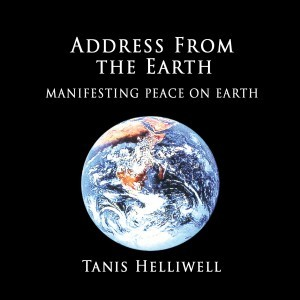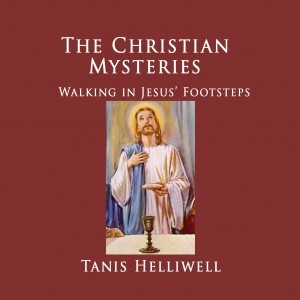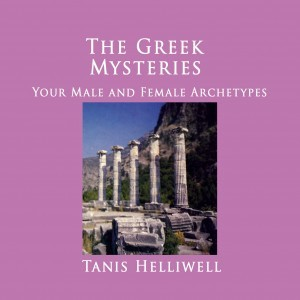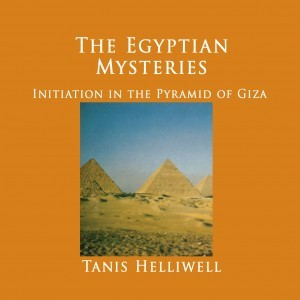▪ The Celtic Mysteries
▪ The Egyptian Mysteries
▪ The Christian Mysteries
▪ The Greek Mysteries
 ▪ The Celtic Mysteries
 ▪ The Egyptian Mysteries
 ▪ The Christian Mysteries
 ▪ The Greek Mysteries
 table div table+table+table+table+table+table+table+table+table+table+table+table+table+table+table+table+table+table+table+table+table+table div table{width:100%;padding:0}table div table+table+table+table+table+table+table+table+table+table+table+table+table+table+table+table+table+table+table+table+table+table div table img{width:96.23%;padding:0;float:none}table div table+table+table+table+table+table+table+table+table+table+table+table+table+table+table+table+table+table+table+table+table+table div table td{width:100%;padding:0 1.88% 18px}/* styles *//* styles */ The Personal Growth Package All CDs \$9 each; MP3 downloads \$5.95 each (sale prices).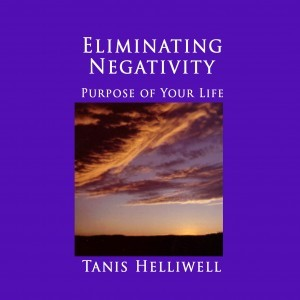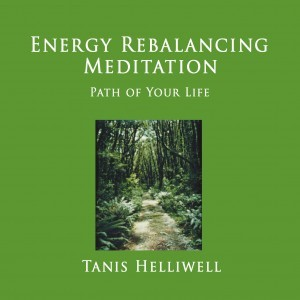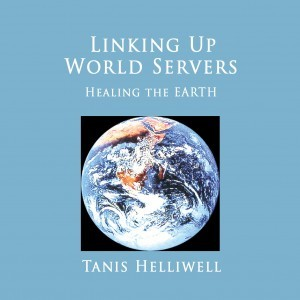▪ Eliminating Negativity
▪ Energy Rebalancing Meditation
 ▪ Eliminating Negativity
 ▪ Energy Rebalancing Meditation
 table div table+table+table+table+table+table+table+table+table+table+table+table+table+table+table+table+table+table+table+table+table+table+table+table+table+table+table div table{width:100%;padding:0}table div table+table+table+table+table+table+table+table+table+table+table+table+table+table+table+table+table+table+table+table+table+table+table+table+table+table+table div table img{width:96.23%;padding:0;float:none}table div table+table+table+table+table+table+table+table+table+table+table+table+table+table+table+table+table+table+table+table+table+table+table+table+table+table+table div table td{width:100%;padding:0 1.88% 18px}/* styles */# Ancestor and Family Healing

Our biological and spiritual ancestors are calling us to heal inherited patterns in our blood and spiritual lineage.

▪ Meet a spiritual ancestor who wants to work with you
▪ Examine core beliefs (scarcity, money or love issues) & heal at source
▪ Experience past life regression to meet key ancient ancestor
▪ Transform past traumas to love others deeply and fully
 ▪ Meet a spiritual ancestor who wants to work with you
 ▪ Examine core beliefs (scarcity, money or love issues) & heal at source
 ▪ Experience past life regression to meet key ancient ancestor
 ▪ Transform past traumas to love others deeply and fully

This course is based on my newest book, The High Beings of Hawaii: My Encounters with Mystical Ancestors. In it, I show how our ancestors influence our lives today and how we can contact them for healing.

 table div table+table+table+table+table+table+table+table+table+table+table+table+table+table+table+table+table+table+table+table+table+table+table+table+table+table+table+table+table+table div table{width:100%;padding:0}table div table+table+table+table+table+table+table+table+table+table+table+table+table+table+table+table+table+table+table+table+table+table+table+table+table+table+table+table+table+table div table img{width:96.23%;padding:0;float:none}table div table+table+table+table+table+table+table+table+table+table+table+table+table+table+table+table+table+table+table+table+table+table+table+table+table+table+table+table+table+table div table td{width:100%;padding:0 1.88% 18px}/* styles */## Photo Album: June workshops in Europe

 /* styles */ I'm now back from my extended travels in Europe, and here are some final memories to share with you. I can't tell you how grateful I am to have shared this time with all of you these last two months. Sweden: My sincere thanks to Olga Karlsson, organizer of the workshop in Stockholm. Olga has also just published Summer with the Leprechauns in Swedish! (Get your copy here!) Germany: Thank you to Christoph Wasser for organizing the annual meditation retreat at Hensellek, Germany - once again one of the most wonderful highlights of our year at IIT! Thanks also to Werner Braun for creating our heart labyrinth and to Irene Ocklenburg for leading the yoga class. Switzerland: Thanks so much to Ingrid Mettier and Jorg Hoffman for organizing the Swiss group.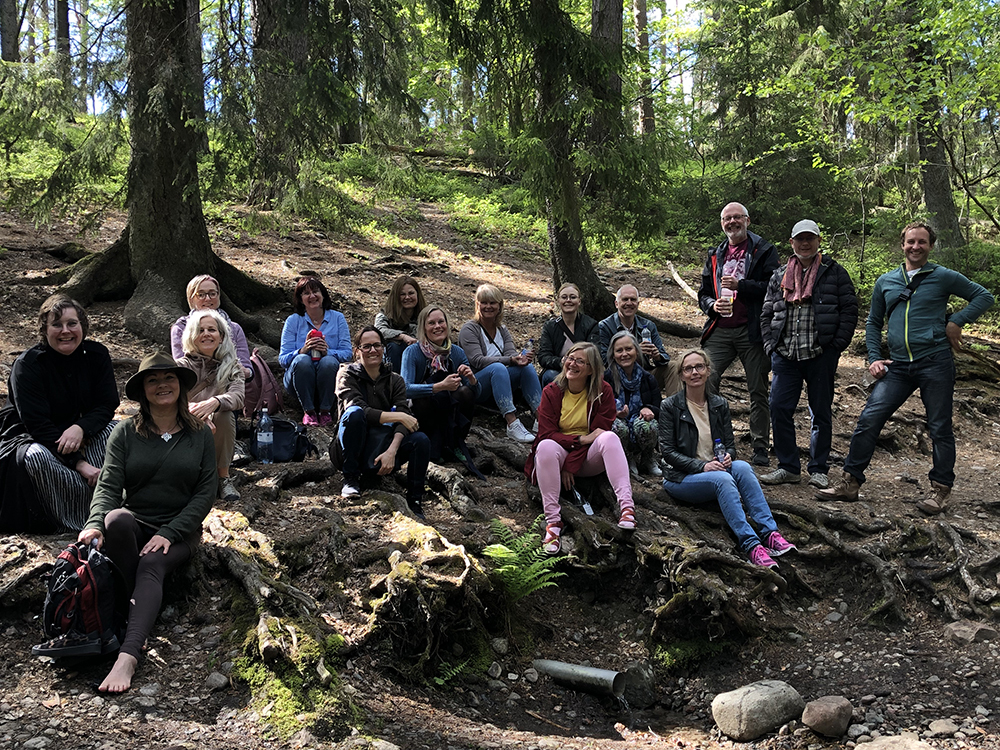The nature spirits workshop group in Sweden.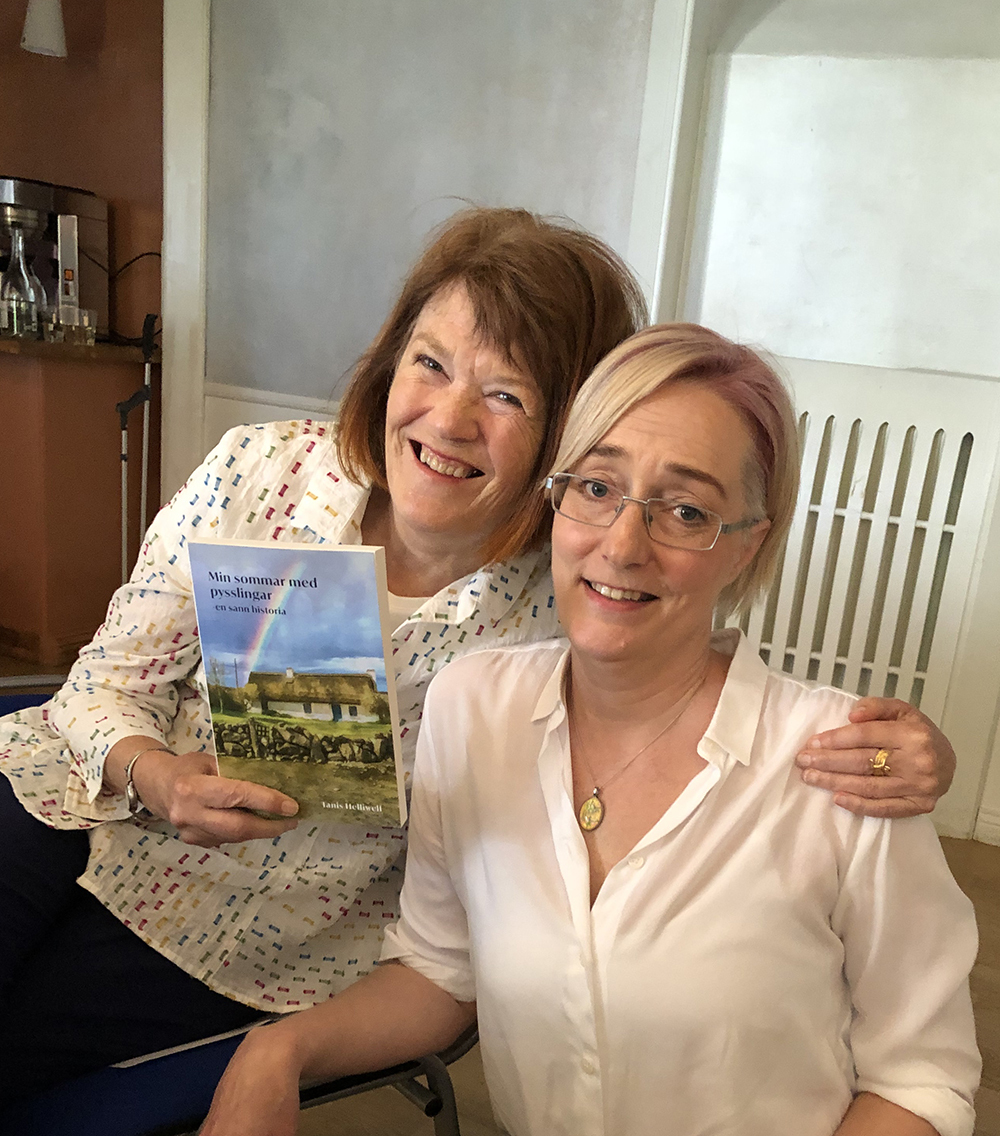Tanis with Olga Karlsson, Swedish workshop organizer.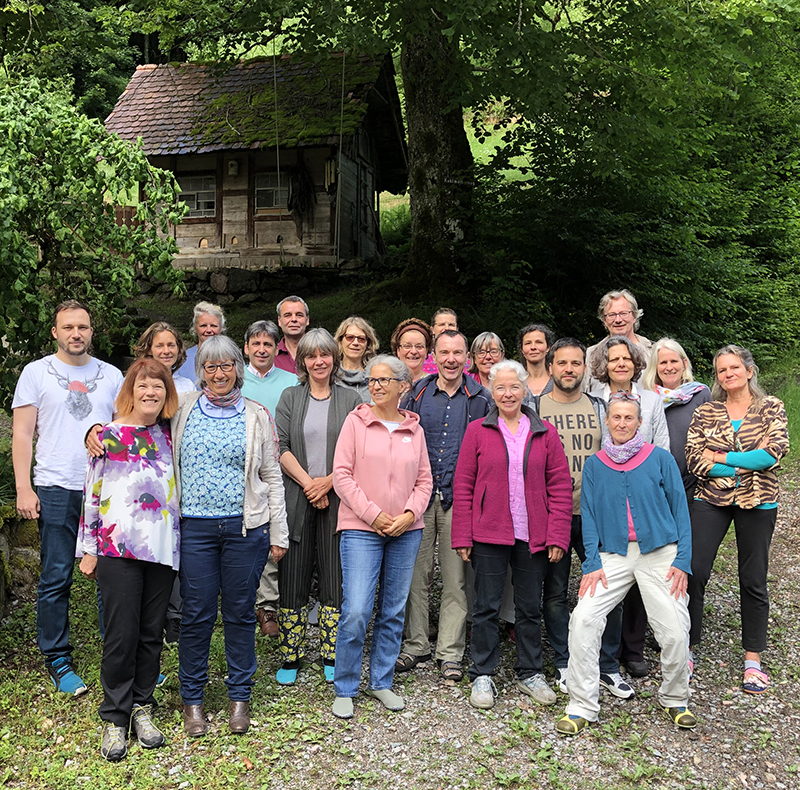The group at the meditation retreat in Hensellek, Germany.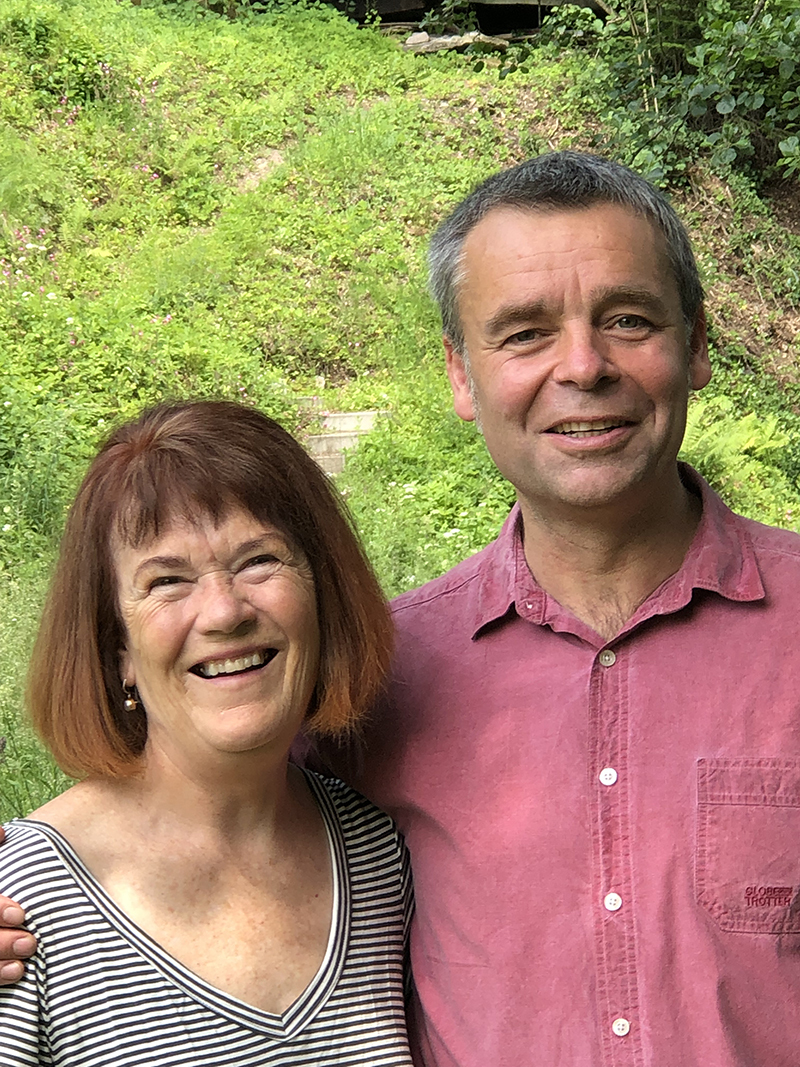Tanis with Hensellek organizer, Christoph Wasser.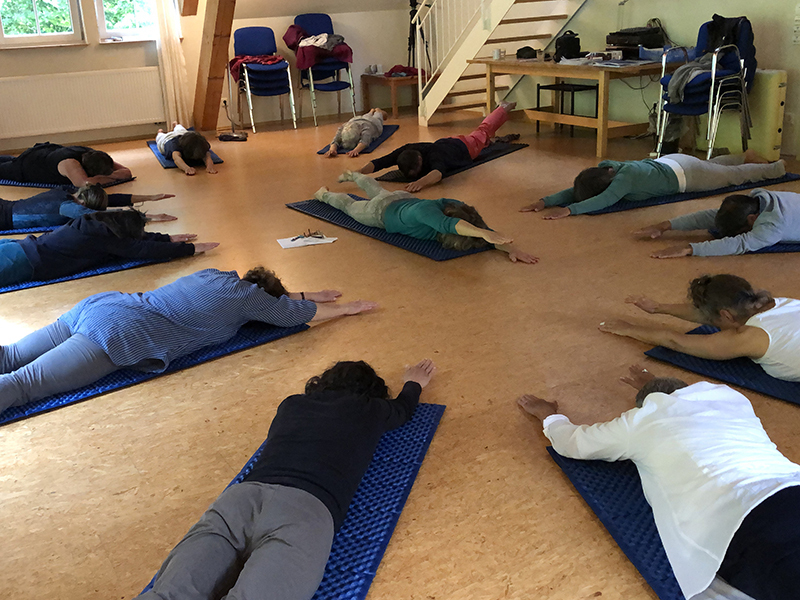Thank you to Irene Ocklenburg for leading the yoga class at Hensellek.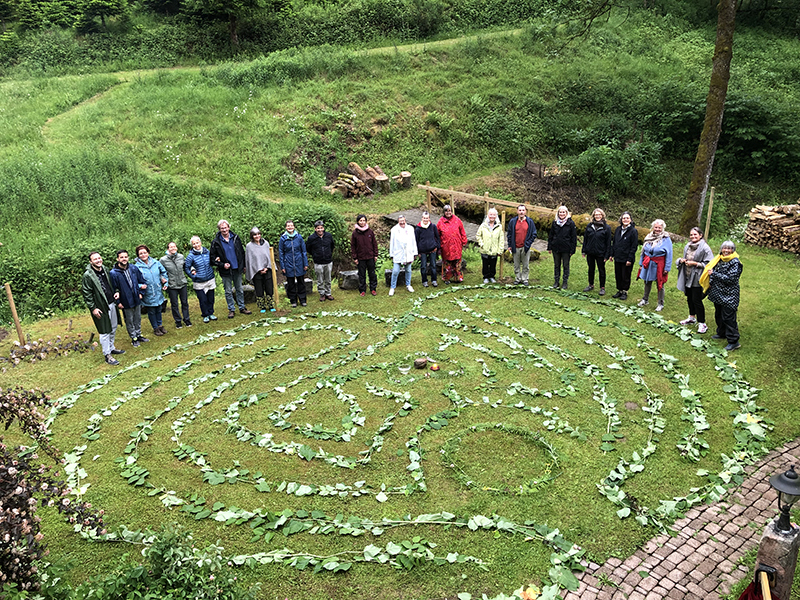Many thanks to Werner Braun for creating a beautiful heart labyrinth for us to walk.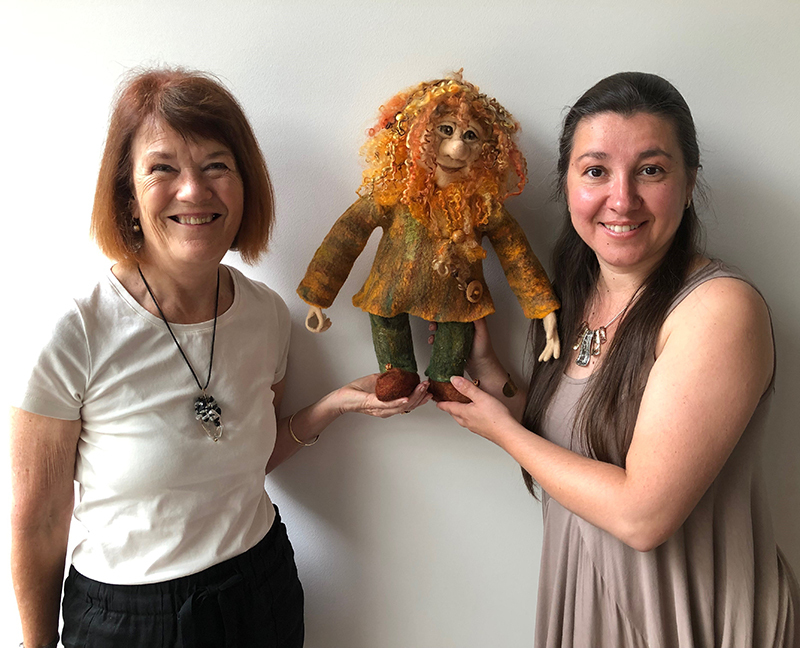Tanis with Lloyd the leprechaun and Monica Slavici…..who made this felt doll of Lloyd!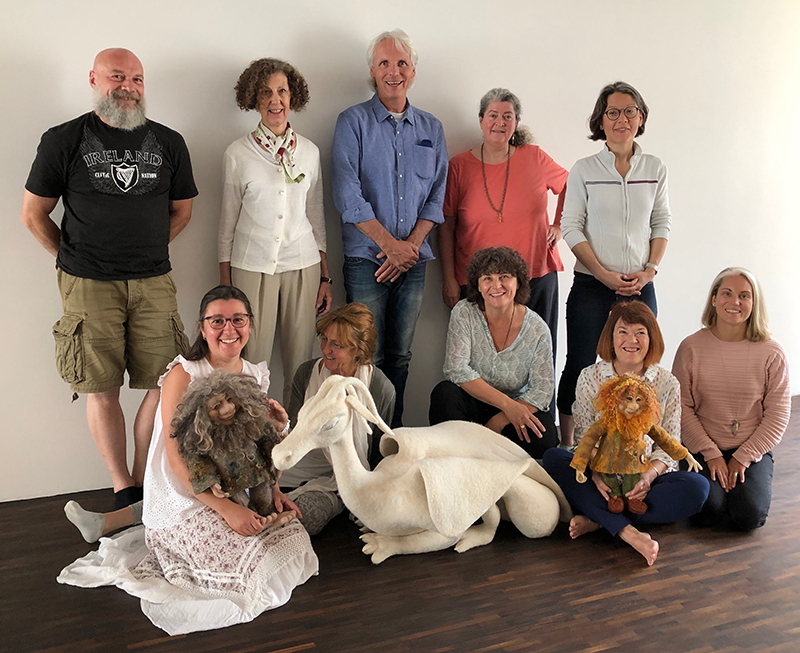The Swiss group.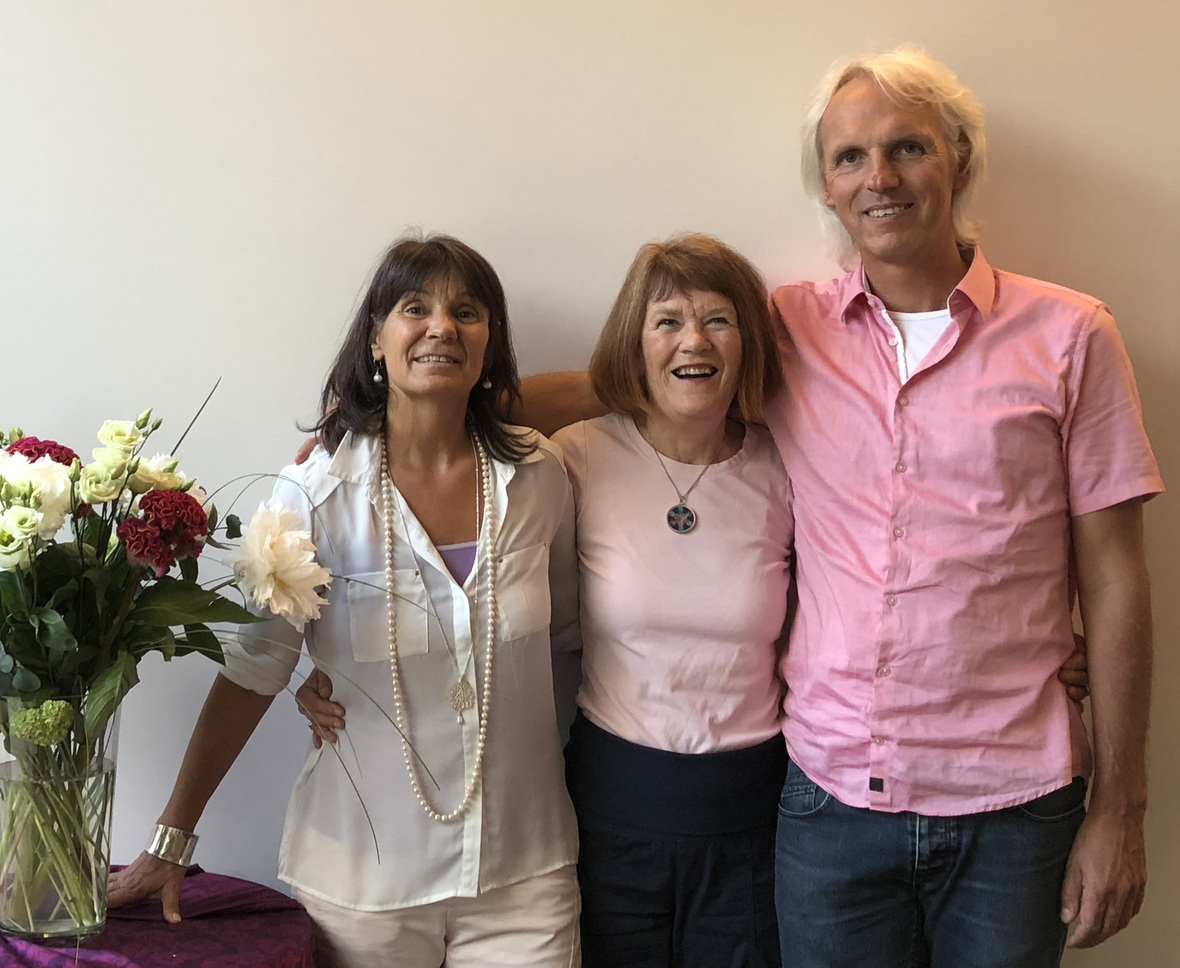Tanis with Ingrid Mettier and Jorg Hoffman in Switzerland.
 table div table+table+table+table+table+table+table+table+table+table+table+table+table+table+table+table+table+table+table+table+table+table+table+table+table+table+table+table+table+table+table+table+table+table+table+table+table+table+table+table+table div table{width:100%;padding:0}table div table+table+table+table+table+table+table+table+table+table+table+table+table+table+table+table+table+table+table+table+table+table+table+table+table+table+table+table+table+table+table+table+table+table+table+table+table+table+table+table+table div table img{width:96.23%;padding:0;float:none}table div table+table+table+table+table+table+table+table+table+table+table+table+table+table+table+table+table+table+table+table+table+table+table+table+table+table+table+table+table+table+table+table+table+table+table+table+table+table+table+table+table div table td{width:100%;padding:0 1.88% 18px}/* styles */# July 14 - 20, 2019 | CANADA | ONLY 1 SPOT LEFT!

## LOVE YOURSELF WELL: Self-healing with the Body Elemental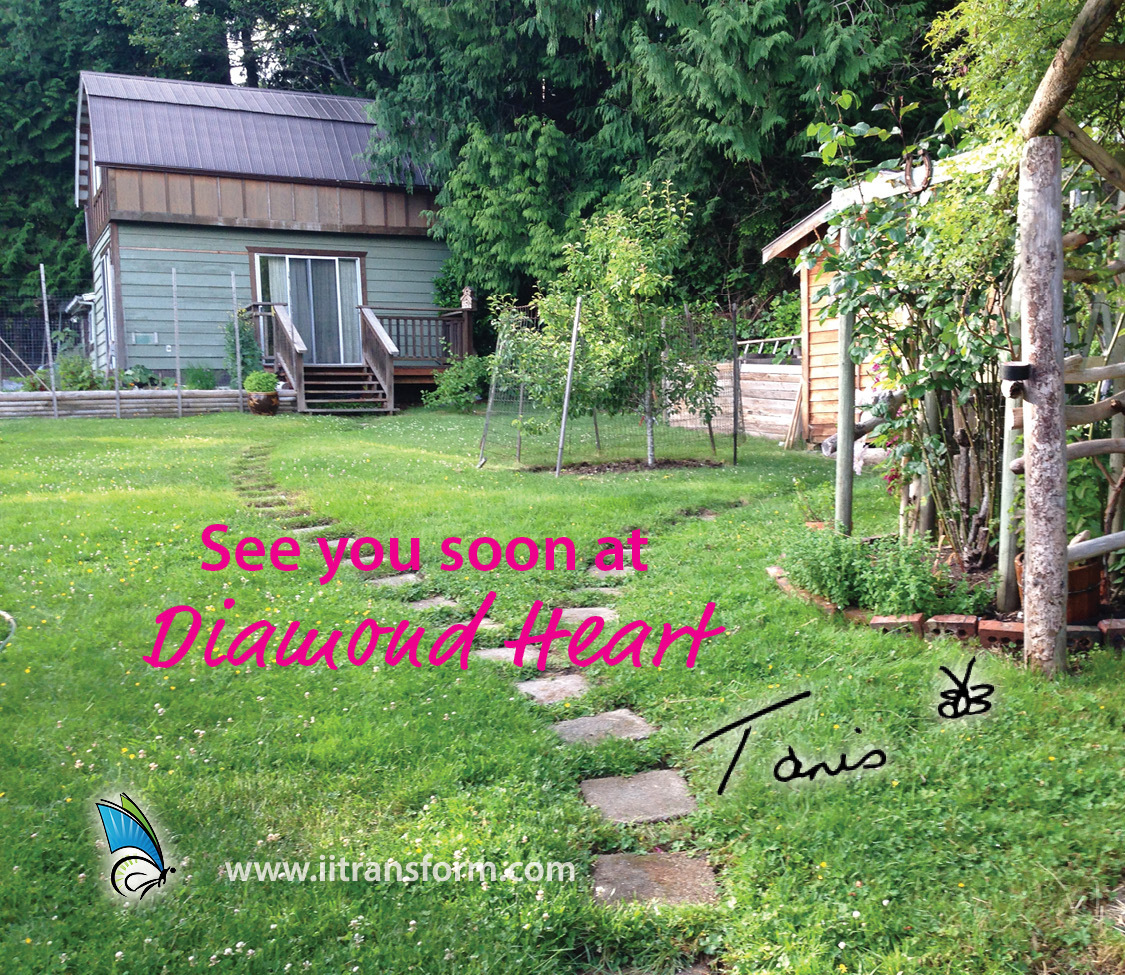There is still one more spot available for the program. Accommodation for this last seat will be only minutes from Diamond Heart, in a cabin by the ocean (accommodation at Diamond Heart itself is now full).

 table div table+table+table+table+table+table+table+table+table+table+table+table+table+table+table+table+table+table+table+table+table+table+table+table+table+table+table+table+table+table+table+table+table+table+table+table+table+table+table+table+table+table+table+table+table div table{width:100%;padding:0}table div table+table+table+table+table+table+table+table+table+table+table+table+table+table+table+table+table+table+table+table+table+table+table+table+table+table+table+table+table+table+table+table+table+table+table+table+table+table+table+table+table+table+table+table+table div table img{width:96.23%;padding:0;float:none}table div table+table+table+table+table+table+table+table+table+table+table+table+table+table+table+table+table+table+table+table+table+table+table+table+table+table+table+table+table+table+table+table+table+table+table+table+table+table+table+table+table+table+table+table+table div table td{width:100%;padding:0 1.88% 18px}/* styles */table div table+table+table+table+table+table+table+table+table+table+table+table+table+table+table+table+table+table+table+table+table+table+table+table+table+table+table+table+table+table+table+table+table+table+table+table+table+table+table+table+table+table+table+table+table+table+table+table+table div table{width:100%;padding:0}table div table+table+table+table+table+table+table+table+table+table+table+table+table+table+table+table+table+table+table+table+table+table+table+table+table+table+table+table+table+table+table+table+table+table+table+table+table+table+table+table+table+table+table+table+table+table+table+table+table div table img{width:96.23%;padding:0;float:none}table div table+table+table+table+table+table+table+table+table+table+table+table+table+table+table+table+table+table+table+table+table+table+table+table+table+table+table+table+table+table+table+table+table+table+table+table+table+table+table+table+table+table+table+table+table+table+table+table+table div table td{width:100%;padding:0 1.88% 18px}/* styles */